# McGraw Hill Math Grade 2 Chapters 1–4 Review Test Answer Key

Practice questions available in McGraw Hill Math Grade 2 Answer Key PDF Chapters 1–4 Review Test will engage students and is a great way of informal assessment.

Add or subtract. Write the sum or difference.

Question 1.
2 + 4 = ____
6

Explanation:
The sum of 2 and 4 is 6.

Question 2.
11 + 3 = ___
14

Explanation:
The sum of 11 and 3 is 14.

Question 3.
9 + 8 = ___
17

Explanation:
The sum of 9 and 8 is 17.

Question 4.
4 + 2 + 7 = ____
13

Explanation:
4 + 2 + 7 = (4 + 2) + 7
= 6 + 7 = 13
So, the sum of 4, 2 and 7 is 13.

Question 5.
7 – 3 = ___
4

Explanation:
The sum of 7 and 3 is 4.

Question 6.
10 – 10 = ___
0

Explanation:
The difference of any number from itself is 0.

Question 7.
18 – 4 = ___
14

Explanation:
The difference of 18 and 4 is 14.

Question 8.
14 – 9 = ____
5

Explanation:
The difference of 14 and 9 is 5.

Solve. Write the number sentence. Then write the answer.

Question 9.
Lucy catches 4. Her mom catches 9. How manydo they catch in all?
____ + ____ = ___4 + 9 = 13Explanation:
Lucy catches 4Her mom catches 94 + 9 = 13
So, they catch 13in all.

Question 10.
Ken has 8. He gives 6 to his brother. Then his dad gives Ken 7 more. How manydoes Ken have now?
____ – ___ = _______ + ___ = ___8 – 6 = 22 + 7 = 9Explanation:
Ken has 8He gives 6 to his brother
Then his dad gives Ken 7 moreFirst subtract: 8 – 6 = 2
Then add: 2 + 7 = 9
So, Ken have 9now.

Question 11.
There are 15on a plate. Annie takes 6. How manyare left?
____ – ____ = ____15 – 6 = 9Explanation:
There are 15on a plate
Annie takes 6Subtract: 15 – 6 = 9
So, 9are left.

Question 12.
A store has 6. It also has 10. The store sells 8 kites. How many kites are left?
___ + ___ = ____ kites
___ – ___ = ____ kites
6 + 10 = 16 kites
16 – 8 = 8 kites

Explanation:
A store has 6It also has 10The store sells 8 kites
Add: 6 + 10 = 16
Subtract: 16 – 8 = 8
So, There are 8 kites left.

Draw a picture and write a number sentence to solve. Use another sheet of paper for your drawing.

Question 13.
Ms. Chan has 11 black buttons. She has 6 brown buttons. How many buttons does she have in all?
____ = ?
______ buttons
11 + 6 = 17
17 buttons

Explanation:
Ms. Chan has 11 black buttons
She has 6 brown buttons
11 + 6 = 17
So, there are 17 buttons in all.

Question 14.
Jill has 14 toy cars. She gives 8 of them to her sister. How many cars does Jill have now?
_______ = ?
___ toy cars
14 – 8 = 6
6 toy cars

Explanation:
Jill has 14 toy cars
She gives 8 of them to her sister
Subtract to find
14 – 8 = 6
So, Jill have 6 toy cars now.

Add or subtract. Write the sum or difference.

Question 15.Explanation:
The sum of 34 and 15 is 49.

Question 16.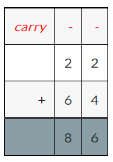Explanation:
The sum of 22 and 64 is 86.

Question 17.Explanation:
The sum of 38 and 27 is 65.

Question 18.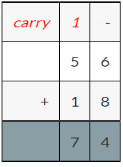Explanation:
The sum of 56 and 18 is 74.

Question 19.Explanation:
The difference of 58 and 23 is 35.

Question 20.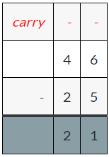Explanation:
The difference of 46 and 25 is 21.

Question 21.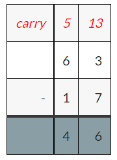Explanation:
The difference of 63 and 17 is 46.

Question 22.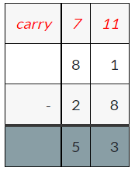Explanation:
The difference of 81 and 28 is 53.

Question 23.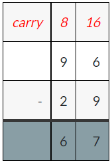Explanation:
The difference of 96 and 29 is 67.

Write the number sentence for each exercise. Then solve and write your answer.

Question 24.
There are 35 books on a shelf. Ms. Jones takes 9 books. How many books are left?
____ books
26 books

Explanation:
There are 35 books on a shelf
Ms. Jones takes 9 books
Subtract to find the number of books left
35 – 9 = 26
So, 26 books are left.

Question 25.
June has 67 blocks. Kevin has 48 blocks. How many more blocks does June have than Kevin?
____ blocks
19 blocks

Explanation:
June has 67 blocks
Kevin has 48 blocks
Subtract to find how many more
67 – 48 = 19
So, June has 19 blocks more than Kevin.

Question 26.
A store has 48 bikes. The store sells 20 bikes. The store gets 35 more bikes. How many bikes are in the store now?
____ bikes
63 bikes

Explanation:
A store has 48 bikes
The store sells 20 bikes
First subtract: 48 – 20 = 28
The store gets 35 more bikes
Now add: 28 + 35 = 63
So, 63 bikes are in the store now.

Question 27.
Mr. Lewis has 83 nails. He uses 56 of them. He gets 30 more nails. How many nails does he have now?
____ nails
57 nails

Explanation:
Mr. Lewis has 83 nails
He uses 56 of them
First subtract: 83 – 56 = 27
He gets 30 more nails
Now add: 27 + 30 = 57
So, Mr. Lewis have 57 nails now.

Question 28.
Bob has 28 stamps. Nick has 53 stamps. They use 47 stamps. How many stamps are left over?
____ stamps
34 stamps

Explanation:
Bob has 28 stamps
Nick has 53 stamps
Add: 28 + 53 = 81
They use 47 stamps
Subtract: 81 – 47 = 34
So, 34 stamps have left over.

Question 29.
A store has a basket with 44 apples in it. Another basket has 19 apples. The store sells 40 apples. How many apples does the store still have?
____ apples
23 apples

Explanation:
A store has a basket with 44 apples in it
Add: 44 + 19 = 63
The store sells 40 apples
Subtract: 63 – 40 = 23
So, there are 23 apples still in the store.

Solve. Write the unknown number.

Question 30.
42 += 70= ___= 28

Explanation:
42 + ? = 70
Subtract to find the unknown addend
70 – 42 = 28
So, 42 + 28 = 70.

Question 31.– 24 = 44= ___= 68

Explanation:
? – 24 = 44
44 + 24 = ?
44 + 24 = 68
The missing number is 68
So, 68 – 24 = 44.

Is the number odd or even? Use objects to help. Put them in pairs. Then circle odd or even.

Question 32.
6 odd evenExplanation:
6 is divisible by 2
So, it is even number.

Question 33.
13 odd even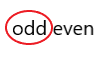Explanation:
13 is not divisible by 2
So, it is an odd number.

Question 34.
9 odd evenExplanation:
9 is not divisible by 2
So, it is an odd number.

Question 35.
18 odd evenExplanation:
18 is divisible by 2
So, it is even number.

Circle pairs of objects. Count by 2s. Write the numbers.

Question 36.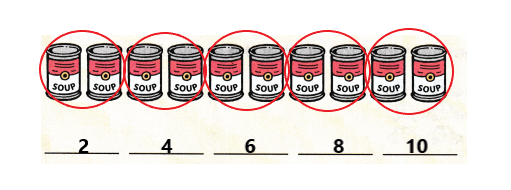Explanation:
I circled pairs of objects
I counted by 2’s and wrote the numbers 2 4 6 8 10
The number increases by 2 each time.

Circle the objects into groups of five. Then count by 5s. Write the numbers.

Question 37.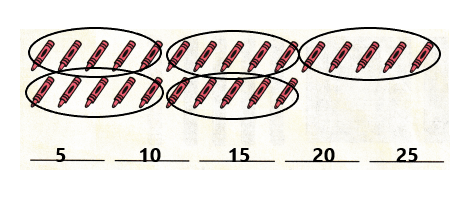Explanation:
I circled pairs of objects
I counted by 5’s and wrote the numbers 5 10 15 20 25
The number increases by 5 each time.

Look at the array. Write the sum.

Question 38.5 + 5 + 5 = ____ cherries
5 + 5 + 5 = 15 cherries

Explanation:
There are 3 rows of 5 cherries in each row
So, 5 + 5 + 5 = 15.

Question 39.4 + 4 = ____ ducks
4 + 4 = 8 ducks

Explanation:
There are 2 rows of 4 ducks in each row
So, 4 + 4 = 8.

Question 40.
4 + 5 = _____
odd evenExplanation:
4 + 5 = 9
The number 9 is not divisible by 2
It is odd number.

Question 41.
8 + 6 = _____
odd evenExplanation:
8 + 6 = 14
The number 14 is divisible by 2
It is even number.

Write a number sentence to tell what the array shows.

Question 42.____ + ___ + ___ = ____
4 + 4 + 4 = 12

Explanation:
There are 3 rows of 4 cars in each row
So, 4 + 4 + 4 = 12
Therefore, there are 12 cars in all.

Count the hundreds, tens, and ones. Write the numbers.

Question 43.There are ___ hundreds, ___ tens, and _____ ones.
There are 5 hundreds 4 tens and 7 ones

Explanation:
There are 2 hundred blocks, 4 tens sticks and 7 ones cubes.

Question 44.
Skip count by 5s. Write the numbers.
245, 250 _____ _____ _____ _____ _____
245, 250, 255, 260, 265, 270, 275

Explanation:
I counted by 5’s and wrote the next 5 numbers from 250
The number increases by 5 each time.

Question 45.
Skip count by 10s. Write the numbers.
300, 310 ____ ____ ____ ____ ____
300, 310, 320, 330, 340, 350

Explanation:
I counted by 10’s and wrote the next 5 numbers from 310
The number increases by 10 each time.

Question 46.
Skip count by 100s. Write the numbers.
300 400 _____ _____ _____ _____ ___
300 400 500 600 700 800 900

Explanation:
I counted by 100’s and wrote the next 5 numbers from 400
The number increases by 100 each time.

Question 47.Write the number shown.
_________
452

Explanation:
There are 4 hundreds 5 tens and 2 ones
So, the number is 452.

Question 48.
Write the number in words.
_________
Four hundred fifty-two

Explanation:
The number 452 in words is Four hundred fifty-two.

Compare each set of numbers. Write <, >, or = to tell how they compare.

Question 49.
460 ____ 535
460 < 535

Explanation:
The number 460 is less than 535.

Question 50.
327 ___ 314
327 > 314

Explanation:
The number 327 is greater than 314.

Question 51.
819 ___ 819
819 = 819

Explanation:
The number 819 is equal to 819.

Question 52.
396 ___ 392